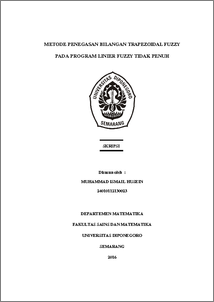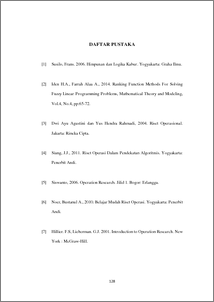# METODE PENEGASAN BILANGAN TRAPEZOIDAL FUZZY PADA PROGRAM LINIER FUZZY TIDAK PENUH

HUSEIN, MUHAMMAD ISMAIL (2016) METODE PENEGASAN BILANGAN TRAPEZOIDAL FUZZY PADA PROGRAM LINIER FUZZY TIDAK PENUH. Undergraduate thesis, UNDIP.Preview
PDF
670KbPDF
Restricted to Repository staff only

2553KbPreview
PDF
282Kb

## Abstract

Fuzzy linear programming is one of linier programming form which there is fuzzy number form on objective variable, objective variable coefficient, constraint coefficient or right hand constraint. Fuzzy linear programming solved by parameters changing which is in fuzzy number form into crisp number form. Crisp method used to averment the trapezoidal fuzzy number and each method is not always has same result. Fuzzy linear programming problem with objective function coefficient, constraint coefficient, or right hand as a fuzzy number is the form of not fully fuzzy linear programming. This paper will discuss three crisp method, they are Ranking Function, Yager Ranking Function, and Robust Ranking Function to solve fuzzy linear programming problem. The final solution can use simplex method, and then final result compared to determine crisp method which is optimal. Keyword : Not fully fuzzy linear programming, trapezoidal fuzzy number, Ranking Function method, Yager Ranking Function method, Robust Ranking Function method, Simplex method.

Item Type: Thesis (Undergraduate) Q Science > QA Mathematics Faculty of Science and Mathematics > Department of Mathematics 84278 INVALID USER 14 Jun 2022 08:57 14 Jun 2022 08:57

Repository Staff Only: item control page